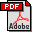Lanczos Algorithm on the Grassmann Manifold
Ana Paula Lopes (ISCAP)
Ana Julia Viamonte (Universidade Portucalense)
Antonio Jose Pascoal (Universidade Portucalense)
Abstract:
In this paper we developed a new Lanczos algorithm on the Grassmann manifold. This work comes in the wake of the article by A. Edelman, T. A. Arias and S. T. Smith, The geometry of algorithms with orthogonality constraints [EAS98], where they presented a new conjugate gradient algorithm on the Grassmann and Stiefel manifolds. These manifolds which are based on orthogonality constraints, yields penetrating insight into many numerical algorithms of linear algebra. They have developed an approach to numerical algorithms involving orthogonality constraints. As the Lanczos method and the method of conjugate gradients are closely related, and one of the main problems of the Lanczos method is the loss of orthogonality, arose the idea of checking whether it would be possible to get a Lanczos algorithm on the Grassmann manifold.
Keywords:
Numerical Algorithms for CS&EDownload the fullpaper
Toulouse | France | 2008 | June | 24  25  26  27